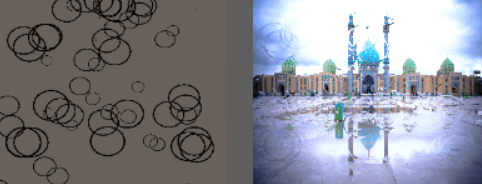How can I make natural rain drops on screen?

I'm trying to make rain effect drop with metaballs and trail on screen.I find a clue in shadertoy but I didn't understand how implemented:

unfortunately it have many math calculations and i can't use it in unity because it produces a lag.obviously I should use textures but how can I have trail effect?!my idea is using a texture and trail renderer for droping but how can I have metaballs effect?Update

https://github.com/smkplus/RainFX/tree/master

but I haven't Idea about trail

I think you should think about the effect as "calculate a map of where the water is" + "generate a normal vector from that map and use it to offset a background texture lookup".

Breaking down what your example shadertoy does, it just calculates a "trail" to show where the defogging happens:Calculates the normals of the circular raindrops,and uses that normal map to offset a texture lookup to fake refraction.

If you want the trails done via post-processing in a shader, you should just create the "trail" shape in the shader using some algebra. For example, in the following function, I've overlaid a "wobbly path" and a taper at the head and tail to get

float trailDrop(vec2 uv, vec2 id, float t) {
// wobbly path
float wobble = 0.5 + 0.5
* cos(120.0 * uv.y)
* sin(50.0 * uv.y);
float v = 1.0 - 10.0 * abs(uv.x - 0.5 + 0.2 * wobble);
v *= clamp(30.0 * uv.y, 0.0, 1.0);
v *= clamp( uv.y + 7.0 * t - 0.6, 0.0, 1.0);
// tail
v *= clamp(1.0 - uv.y - pow(t, 2.0), 0.0, 1.0);
return clamp(v * 10.0, 0.0, 1.0);
}

Here's a rough POC in shadertoy -- https://www.shadertoy.com/view/XlBfz1 demonstrating creating a set of raindrop trails. It looks grainy at small resolutions due to the derivatives resolution but should look better if you fullscreen it.Left as an exercise to the reader:

1) Add the small round drops. for inspiration, look at the StaticDrops function in your original shadertoy example.

2) Add in high-quality normal calculations. As the #define CHEAP_NORMALS option in your original shadertoy example implies, the builtin dFdx is a low-fidelity approximation and you can get better results by manually calculating the derivatives (at the cost of calculating the function 3 times).

3) Randomizing the spacing between the columns. You can widen the columns and then modify the uv.x - 0.5 + 0.2 * wobble bit to add a random offset on the x axis. You'll also probably want to take a page out of the original example once again and layer a couple different sized layers of streams on top of each other to get a less uniform look.

you can make this effect by following the steps below:

Particle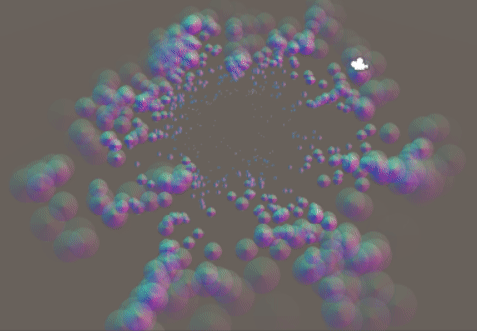RenderTextuer

you can store result by using RenderTexture. this is example of multipass in shadertoy:

iñigo quilez: Shadertoy uses multiple passes, one per "Buffer". As the name indicates, this passes store the results in a buffer. A buffer is just a texture. Unity will let you render to textures too.

I created a camera to Rendering particles to RenderTexture: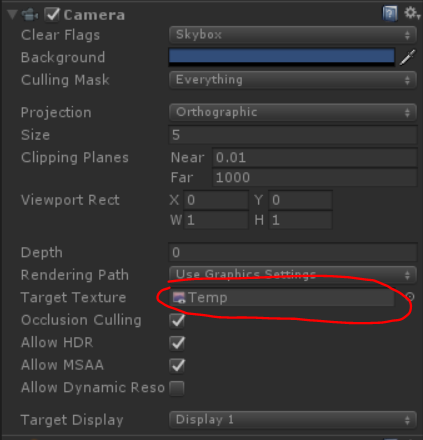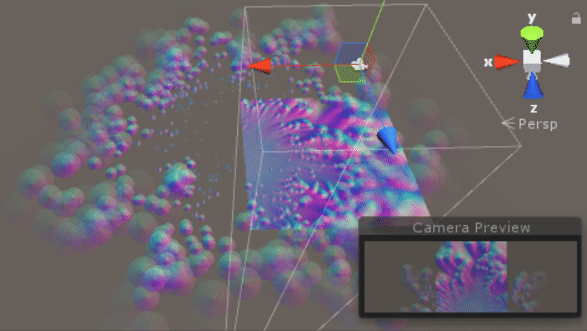GrabPassing

you can grab pass for applying Distortion

I explained it in this post:

How can I replicate Quantum Break's distortion particle effect?

Blur

by using alpha in color over Lifetime we have simple blur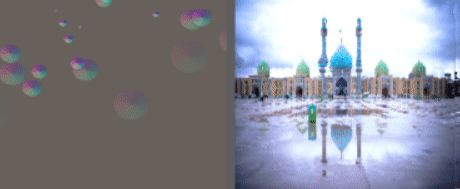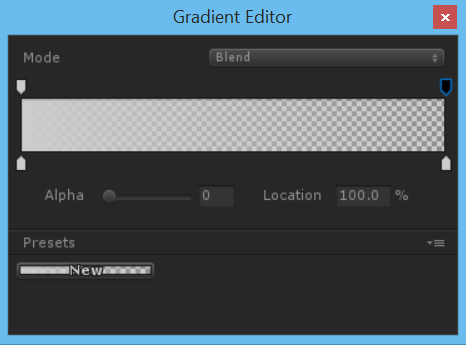to get better result it's better to use simple blur , but how we achive blur?

Convolution matrix

In image processing, a kernel, convolution matrix, or mask is a small matrix. It is used for blurring, sharpening, embossing, edge detection, and more. This is accomplished by doing a convolution between a kernel and an image.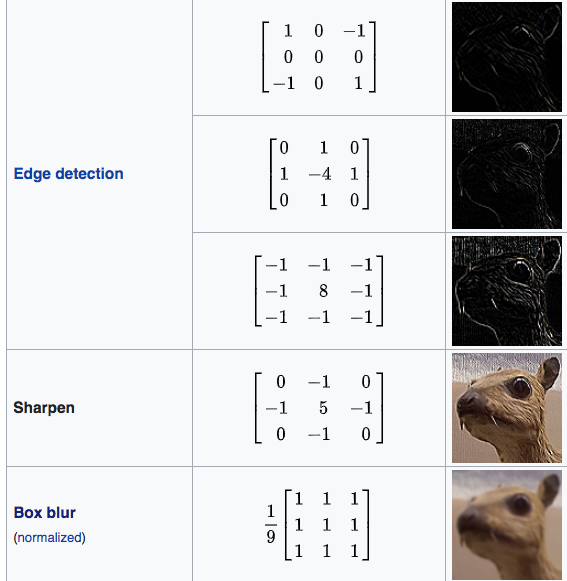{
Properties
{
_MainTex ("Texture", 2D) = "white" {}
[Enum(kerEdgeDetectionA,1,kerEdgeDetectionB,2,kerEdgeDetectionC,3,kerSharpen,4,kerBoxBlur,5)]
_Kernel("Kernel", Float) = 1
}
{
// No culling or depth
Cull Off ZWrite Off ZTest Always

Pass
{
CGPROGRAM

#pragma vertex vert
#pragma fragment frag

#include "UnityCG.cginc"

struct appdata
{
float4 vertex : POSITION;
float2 uv : TEXCOORD0;
};

struct v2f
{
float2 uv : TEXCOORD0;
float4 vertex : SV_POSITION;
};

v2f vert (appdata v)
{
v2f o;
o.vertex = UnityObjectToClipPos(v.vertex);
o.uv = v.uv;
return o;
}

sampler2D _MainTex;
float4 _MainTex_TexelSize;

float3x3 GetData(int channel, sampler2D tex, float2 uv, float4 size)
{
float3x3 mat;
for (int y=-1; y<2; y++)
{
for(int x=-1; x<2; x++)
{
mat[x+1][y+1]=tex2D(tex, uv + float2(x*size.x, y*size.y))[channel];
}
}
return mat;
}
float3x3 GetMean(float3x3 matr, float3x3 matg, float3x3 matb)
{
float3x3 mat;
for (int y=0; y<3; y++)
{
for(int x=0; x<3; x++)
{
mat[x][y] = (matr[x][y] + matg[x][y] + matb[x][y]) / 3.0;
}
}
return mat;
}

float Convolve(float3x3 kernel, float3x3 pixels, float denom, float offset)
{
float res = 0.0;
for (int y=0; y<3; y++)
{
for(int x=0; x<3; x++)
{
res += kernel[2-x][2-y]*pixels[x][y];
}
}

return  res;
}

float _Kernel;

fixed4 frag (v2f i) : SV_Target
{

float3x3 kerEdgeDetectionA = float3x3 (    0.0,  0,  -1.0,
1.0,  0,  -1.0,
0.0,  1.0,  0.0);

float3x3 kerEdgeDetectionB = float3x3 (0.0,  1.0,  0.0,
1.0, -4.0,  1.0,
0.0,  1.0, 0.0);

float3x3 kerEdgeDetectionC = float3x3 (-1.0, -1.0, -1.0,
-1.0,  8.0, -1.0,
-1.0, -1.0, -1.0);

float3x3 kerSharpen = float3x3 (0.0, -1.0, 0.0,
-1.0, 5.0, -1.0,
0.0, -1.0, 0.0);

float3x3 kerBoxBlur = (1.0/9.0)*float3x3 (    1.0,  1.0,  1.0,
1.0,  1.0,  1.0,
1.0,  1.0,  1.0);

float3x3 kernelSelection;
if(_Kernel == 1){
kernelSelection = kerEdgeDetectionA;
}else if(_Kernel == 2){
kernelSelection = kerEdgeDetectionB;
}else if(_Kernel == 3){
kernelSelection = kerEdgeDetectionC;
}else if(_Kernel == 4){
kernelSelection = kerSharpen;
}else if(_Kernel == 5){
kernelSelection = kerBoxBlur;
}

float3x3 matr = GetData(0, _MainTex, i.uv, _MainTex_TexelSize);
float3x3 matg = GetData(1, _MainTex, i.uv, _MainTex_TexelSize);
float3x3 matb = GetData(2, _MainTex, i.uv, _MainTex_TexelSize);
float3x3 mata = GetMean(matr, matg, matb);

// kernel
float4 gl_FragColor = float4(Convolve(kernelSelection,matr,1.0,0.0),
Convolve(kernelSelection,matg,1.0,0.0),
Convolve(kernelSelection,matb,1.0,0.0),
1.0);

return gl_FragColor;
}
ENDCG
}
}
}

Boxblur

A box blur (also known as a box linear filter) is a spatial domain linear filter in which each pixel in the resulting image has a value equal to the average value of its neighboring pixels in the input image. It is a form of low-pass ("blurring") filter. A 3 by 3 box blur can be written as matrix

https://en.wikipedia.org/wiki/Box_blur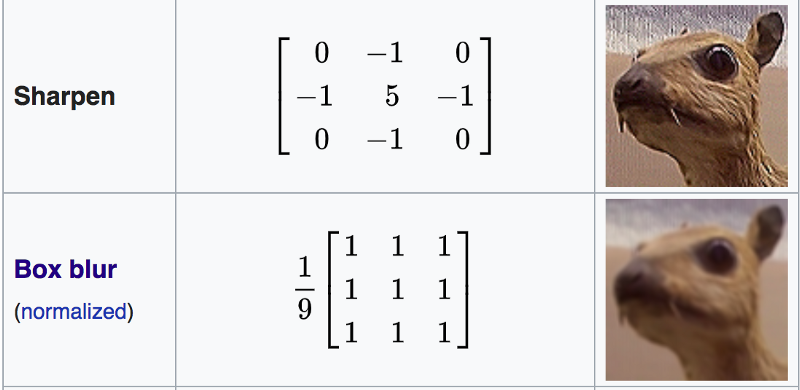{
Properties
{
_MainTex ("Texture", 2D) = "white" {}
}
{
Blend SrcAlpha OneMinusSrcAlpha

Pass
{
CGPROGRAM
#pragma vertex vert
#pragma fragment frag

#include "UnityCG.cginc"

struct appdata
{
float4 vertex : POSITION;
float2 uv : TEXCOORD0;
};

struct v2f
{
float2 uv : TEXCOORD0;
float4 vertex : SV_POSITION;
};

v2f vert (appdata v)
{
v2f o;
o.vertex = UnityObjectToClipPos(v.vertex);
o.uv = v.uv;
return o;
}

sampler2D _MainTex;
float4 _MainTex_TexelSize;

float4 box(sampler2D tex, float2 uv, float4 size)
{
float4 c = tex2D(tex, uv + float2(-size.x, size.y)) + tex2D(tex, uv + float2(0, size.y)) + tex2D(tex, uv + float2(size.x, size.y)) +
tex2D(tex, uv + float2(-size.x, 0)) + tex2D(tex, uv + float2(0, 0)) + tex2D(tex, uv + float2(size.x, 0)) +
tex2D(tex, uv + float2(-size.x, -size.y)) + tex2D(tex, uv + float2(0, -size.y)) + tex2D(tex, uv + float2(size.x, -size.y));

return c / 9;
}

float4 frag (v2f i) : SV_Target
{
float4 col = box(_MainTex, i.uv, _MainTex_TexelSize);
return col;
}
ENDCG
}
}
}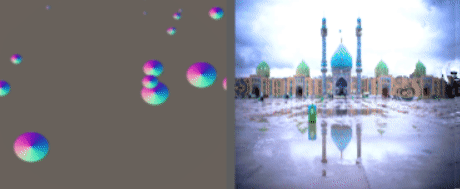Repetition

you can use Rendertexture to store previous frame.so you can grab previous frame then blur. by repeating this you achieve blur.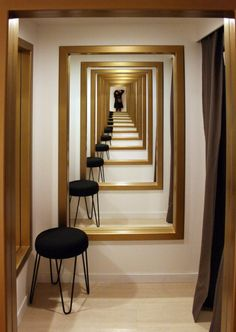Normal

float4 distortion = tex2D(_MainTex,i.uv);
float3 distortionNormal = UnpackNormal(distortion);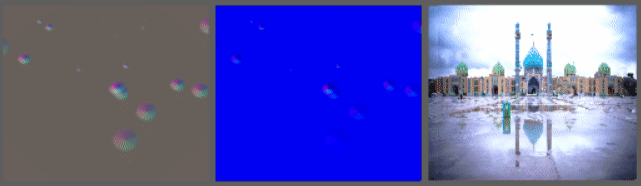Conclusion

{
Properties{
_MainTex("MainTex",2D) = "white"{}
_NormalIntensity("NormalIntensity",Float) = 1
_Alpha("Alpha",Float) = 1
}
{
Tags {"Queue"="Transparent" "RenderType"="Transparent"}
Blend SrcAlpha OneMinusSrcAlpha

GrabPass
{
"_GrabTexture"
}

Pass
{
CGPROGRAM
#pragma vertex vert
#pragma fragment frag

#include "UnityCG.cginc"

struct appdata
{
float4 vertex : POSITION;
float2 uv : TEXCOORD0;
float2 grabPos : TEXCOORD1;
float3 normal :NORMAL;
};

struct v2f
{
float2 uv : TEXCOORD0;
float4 grabPos : TEXCOORD1;
half3 worldNormal :TEXCOORD2;
float4 vertex : SV_POSITION;

};
sampler2D _MainTex;
float _Intensity,_Alpha;

v2f vert (appdata v)
{
v2f o;
o.uv = v.uv;
o.vertex = UnityObjectToClipPos(v.vertex);
o.grabPos = ComputeGrabScreenPos(o.vertex);
o.worldNormal = UnityObjectToWorldNormal(v.normal);
return o;
}

sampler2D _GrabTexture;
float _NormalIntensity;

fixed4 frag (v2f i) : SV_Target
{
float4 distortion = tex2D(_MainTex,i.uv);
float3 distortionNormal = UnpackNormal(distortion);
distortionNormal.xy *= _NormalIntensity;
normalize(distortionNormal);
fixed4 col = tex2Dproj(_GrabTexture, i.grabPos+float4(distortionNormal.rgb,0));
return col;
}
ENDCG
}
}
}

without using alpha in color over Lifetime: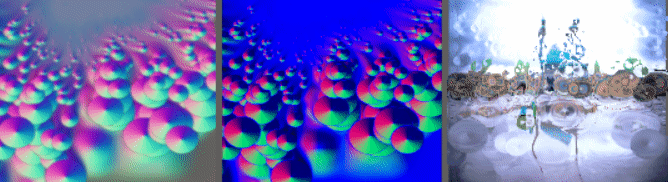by using alpha in color over Lifetime: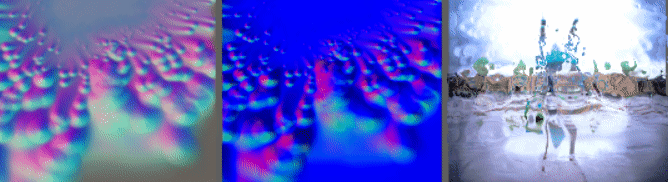Source is available:

https://github.com/smkplus/RainDrop

There's more!

also you can make Ripples